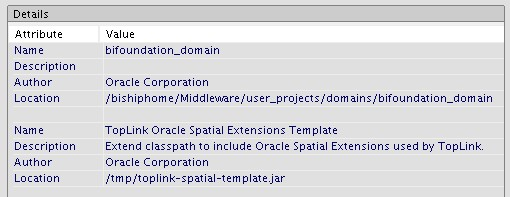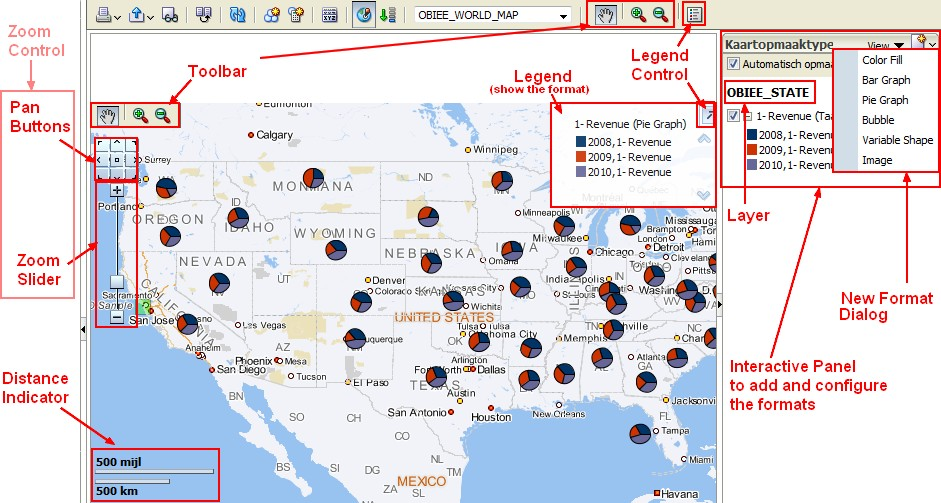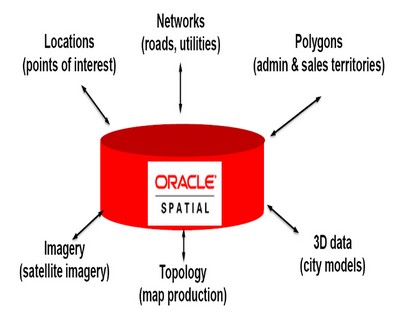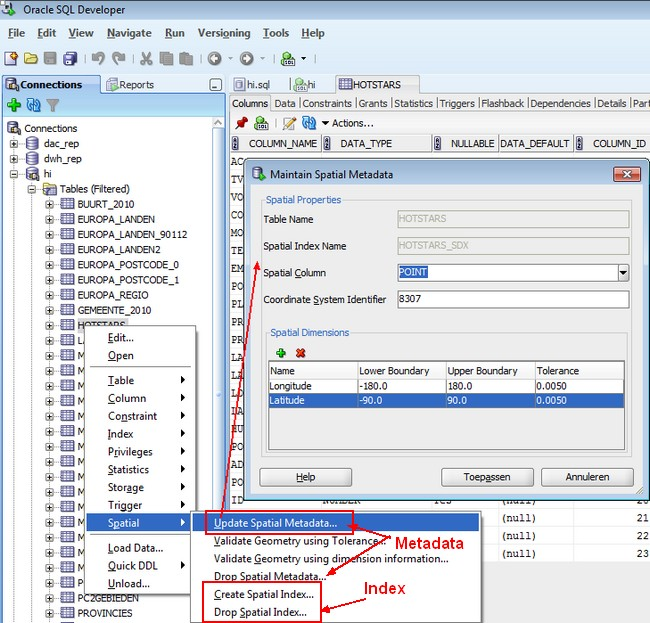# Oracle Spatial - The geometric description (SDO_GEOMETRY DataType)

With Spatial, the geometric description of a spatial object is stored:

• in a single row,
• in a single column of object type SDO_GEOMETRY
• in a user-defined table
• where all geometry share the same coordinate system (sdo_srid attribute)

Any table that has a column of type SDO_GEOMETRY must have another column, or set of columns, that defines a unique primary key for that table. Tables of this sort are sometimes referred to as spatial tables or spatial geometry tables.

## SDO_GEOMETRY

Oracle Spatial defines the object type SDO_GEOMETRY as:

``````CREATE TYPE sdo_geometry AS OBJECT (
SDO_GTYPE NUMBER,
SDO_SRID NUMBER,
SDO_POINT SDO_POINT_TYPE,
SDO_ELEM_INFO SDO_ELEM_INFO_ARRAY,
SDO_ORDINATES SDO_ORDINATE_ARRAY);```
```

where:

• SDO_GTYPE determine the type of the geometry (point, line, polygon, …)
• SDO_SRID determine the coordinate system
• SDO_POINT define the coordinates for a point geometry
• SDO_ORDINATES is an array that store the coordinate values that make up the boundary of the geometry
• SDO_ELEM_INFO gives information on how to interpret the ordinates

### SDO_GTYPE

The SDO_GTYPE attribute indicates the type of the geometry and is 4 digits in the format dltt, where:

• d identifies the number of dimensions (2, 3, or 4)
• l identifies the linear referencing measure dimension for a three-dimensional linear referencing system (LRS) geometry
• tt identifies the geometry type (00 through 07, with 08 through 99 reserved for future use).

The number of dimensions reflects the number of ordinates used to represent each vertex. Points and lines are considered two-dimensional objects.

For example in the Value column of the table below, an SDO_GTYPE value of 2003 indicates a two-dimensional polygon.

Valid SDO_GTYPE Values

Value Geometry Type Description
dl00 UNKNOWN_GEOMETRY Spatial ignores this geometry
dl01 Oracle Spatial - Point Geometry contains one point
dl02 LINE or CURVE Geometry contains one line string that can contain straight or circular arc segments, or both. (LINE and CURVE are synonymous in this context.)
dl03 POLYGON Geometry contains one polygon with or without holes. For a polygon with holes, enter the exterior boundary first, followed by any interior boundaries.
dl04 COLLECTION Geometry is a heterogeneous collection of elements. COLLECTION is a superset that includes all other types.
dl05 MULTIPOINT Geometry has one or more points. (MULTIPOINT is a superset of POINT.)
dl06 MULTILINE or MULTICURVE Geometry has one or more line strings. (MULTILINE and MULTICURVE are synonymous in this context, and each is a superset of both LINE and CURVE.)
dl07 MULTIPOLYGON Geometry can have multiple, disjoint polygons (more than one exterior boundary). (MULTIPOLYGON is a superset of POLYGON.)

In any given layer (column), all geometries must have the same number of dimensions. For example, you cannot mix two-dimensional and three-dimensional data in the same layer (column).

The following methods are available for returning the individual dltt components of the SDO_GTYPE for a geometry object:

• Get_Dims,
• Get_LRS_Dim,
• Get_Gtype.

### SDO_SRID

The SDO_SRID attribute can be used to identify a coordinate system (spatial reference system) to be associated with the geometry.

If SDO_SRID is null, no coordinate system is associated with the geometry.

If SDO_SRID is not null, it must contain a value from the SRID column of the SDO_COORD_REF_SYS table, and this value must be inserted into the SRID column of the USER_SDO_GEOM_METADATA view.

All geometries in a geometry column must have the same SDO_SRID value.

### SDO_POINT

The SDO_POINT attribute is defined using the SDO_POINT_TYPE object type, which has the attributes X, Y, and Z, all of type NUMBER.

If the SDO_ELEM_INFO and SDO_ORDINATES arrays are both null, and the SDO_POINT attribute is non-null, then the X and Y values are considered to be the coordinates for a point geometry. Otherwise, the SDO_POINT attribute is ignored by Spatial. You should store point geometries in the SDO_POINT attribute for optimal storage; and if you have only point geometries in a layer, it is strongly recommended that you store the point geometries in the SDO_POINT attribute.

### SDO_ORDINATES

The SDO_ORDINATES attribute is defined using a varying length array (1048576) of NUMBER type that stores the coordinate values that make up the boundary of a spatial object.

This array must always be used in conjunction with the SDO_ELEM_INFO varying length array. The values in the array are ordered by dimension. For example :

• a polygon whose boundary has four two-dimensional points is stored as {X1, Y1, X2, Y2, X3, Y3, X4, Y4, X1, Y1}.
• if the points are three-dimensional, then they are stored as {X1, Y1, Z1, X2, Y2, Z2, X3, Y3, Z3, X4, Y4, Z4, X1, Y1, Z1}.

Spatial index creation, operators, and functions ignore the Z values because this release of the product supports only two-dimensional spatial objects. The number of dimensions associated with each point is stored as metadata in the xxx_SDO_GEOM_METADATA views, described in Section 2.6.

The values in the SDO_ORDINATES array must all be valid and non-null.

There are no special values used to delimit elements in a multielement geometry. The start and end points for the sequence describing a specific element are determined by the STARTING_OFFSET values for that element and the next element in the SDO_ELEM_INFO array, as explained in Section 2.2.4. The offset values start at 1. SDO_ORDINATES(1) is the first ordinate of the first point of the first element.

### SDO_ELEM_INFO

This attribute lets you know how to interpret the ordinates stored in the sdo_ordinates attribute. The SDO_ELEM_INFO attribute is defined using a varying length array of numbers.

Each triplet set of numbers is interpreted as follows:

#### SDO_STARTING_OFFSET

Indicates the offset within the SDO_ORDINATES array where the first ordinate for this element is stored. Offset values start at 1 and not at 0. Thus, the first ordinate for the first element will be at SDO_GEOMETRY.SDO_ORDINATES(1). If there is a second element, its first ordinate will be at SDO_GEOMETRY.SDO_ORDINATES(n), where n reflects the position within the SDO_ORDINATE_ARRAY definition (for example, 19 for the 19th number, as in Figure 2-3 in Section 2.5.2).

#### SDO_ETYPE

Indicates the type of the element.

Simple elements

SDO_ETYPE values 1, 2, 1003, and 2003 are considered simple elements. They are defined by a single triplet entry in the SDO_ELEM_INFO array. For SDO_ETYPE values 1003 and 2003, the first digit indicates exterior (1) or interior (2):

• 1003: exterior polygon ring (must be specified in counterclockwise order)
• 2003: interior polygon ring (must be specified in clockwise order)

The use of 3 as an SDO_ETYPE value for polygon ring elements in a single geometry is discouraged. You should specify 3 only if you do not know if the simple polygon is exterior or interior, and you should then upgrade the table or layer to the current format using the SDO_MIGRATE.TO_CURRENT procedure, described in Chapter 17

You cannot mix 1-digit and 4-digit SDO_ETYPE values in a single geometry. If you use 4-digit SDO_ETYPE values, you must use 4-digit SDO_GTYPE values.

Compound elements

SDO_ETYPE values 4, 1005, and 2005 are considered compound elements.

They contain at least one header triplet with a series of triplet values that belong to the compound element. For SDO_ETYPE values 1005 and 2005, the first digit indicates exterior (1) or interior (2):

• 1005: exterior polygon ring (must be specified in counterclockwise order)
• 2005: interior polygon ring (must be specified in clockwise order)

The use of 5 as an SDO_ETYPE value for polygon ring elements in a single geometry is discouraged. You should specify 5 only if you do not know if the compound polygon is exterior or interior, and you should then upgrade the table or layer to the current format using the SDO_MIGRATE.TO_CURRENT procedure, described in Chapter 17.

You cannot mix 1-digit and 4-digit SDO_ETYPE values in a single geometry. If you use 4-digit SDO_ETYPE values, you must use 4-digit SDO_GTYPE values.

The elements of a compound element are contiguous. The last point of a subelement in a compound element is the first point of the next subelement. The point is not repeated.

#### SDO_INTERPRETATION

Means one of two things, depending on whether or not SDO_ETYPE is a compound element.

• If SDO_ETYPE is a compound element (4, 1005, or 2005), this field specifies how many subsequent triplet values are part of the element.
• If the SDO_ETYPE is not a compound element (1, 2, 1003, or 2003), the interpretation attribute determines how the sequence of ordinates for this element is interpreted. For example, a line string or polygon boundary may be made up of a sequence of connected straight line segments or circular arcs.

Descriptions of valid SDO_ETYPE and SDO_INTERPRETATION value pairs are given in Table 2-2.

If a geometry consists of more than one element, then the last ordinate for an element is always one less than the starting offset for the next element. The last element in the geometry is described by the ordinates from its starting offset to the end of the SDO_ORDINATES varying length array.

For compound elements (SDO_ETYPE values 4, 1005, or 2005), a set of n triplets (one for each subelement) is used to describe the element.

It is important to remember that subelements of a compound element are contiguous. The last point of a subelement is the first point of the next subelement. For subelements 1 through n-1, the end point of one subelement is the same as the starting point of the next subelement. The starting point for subelements 2…n-2 is the same as the end point of subelement 1…n-1. The last ordinate of subelement n is either the starting offset minus 1 of the next element in the geometry, or the last ordinate in the SDO_ORDINATES varying length array.

The current size of a varying length array can be determined by using the function varray_variable.Count in PL/SQL or OCICollSize in the Oracle Call Interface (OCI).

The semantics of each SDO_ETYPE element and the relationship between the SDO_ELEM_INFO and SDO_ORDINATES varying length arrays for each of these SDO_ETYPE elements are given in Table 2-2.

Table 2-2 Values and Semantics in SDO_ELEM_INFO

SDO_ETYPE SDO_INTERPRETATION Meaning Description
0 (any numeric value) Type 0 (zero) element Used to model geometry types not supported by Oracle Spatial
1 1 Point type
1 0 Orientation for an oriented point
1 n > 1 Point cluster with n points
2 1 Line string whose vertices are connected by straight line segments
2 2 Line string made up of a connected sequence of circular arcs. Each circular arc is described using three coordinates: the start point of the arc, any point on the arc, and the end point of the arc. The coordinates for a point designating the end of one arc and the start of the next arc are not repeated. For example, five coordinates are used to describe a line string made up of two connected circular arcs. Points 1, 2, and 3 define the first arc, and points 3, 4, and 5 define the second arc, where point 3 is only stored once.
1003 or 2003 1 Simple polygon whose vertices are connected by straight line segments. You must specify a point for each vertex, and the last point specified must be exactly the same point as the first (to close the polygon), regardless of the tolerance value. For example, for a 4-sided polygon, specify 5 points, with point 5 the same as point 1.
1003 or 2003 2 Polygon made up of a connected sequence of circular arcs that closes on itself. The end point of the last arc is the same as the start point of the first arc. Each circular arc is described using three coordinates: the start point of the arc, any point on the arc, and the end point of the arc. The coordinates for a point designating the end of one arc and the start of the next arc are not repeated. For example, five coordinates are used to describe a polygon made up of two connected circular arcs. Points 1, 2, and 3 define the first arc, and points 3, 4, and 5 define the second arc. The coordinates for points 1 and 5 must be the same (tolerance is not considered), and point 3 is not repeated.
1003 or 2003 3 Rectangle type (sometimes called optimized rectangle). A bounding rectangle such that only two points, the lower-left and the upper-right, are required to describe it. The rectangle type can be used with geodetic or non-geodetic data. However, with geodetic data, use this type only to create a query window (not for storing objects in the database). For detailed information about using this type with geodetic data, including examples, see Section 6.2.3.
1003 or 2003 4 Circle type Described by three distinct non-colinear points, all on the circumference of the circle
4 n > 1 Compound line string with some vertices connected by straight line segments and some by circular arcs. The value n in the Interpretation column specifies the number of contiguous subelements that make up the line string. The next n triplets in the SDO_ELEM_INFO array describe each of these subelements. The subelements can only be of SDO_ETYPE 2. The last point of a subelement is the first point of the next subelement, and must not be repeated. See Section 2.5.3 and Figure 2-4 for an example of a compound line string geometry.
1005 or 2005 n > 1 Compound polygon with some vertices connected by straight line segments and some by circular arcs. The value n in the Interpretation column specifies the number of contiguous subelements that make up the polygon. The next n triplets in the SDO_ELEM_INFO array describe each of these subelements. The subelements can only be of SDO_ETYPE 2. The end point of a subelement is the start point of the next subelement, and it must not be repeated. The start and end points of the polygon must be exactly the same point (tolerance is ignored). See Section 2.5.4 and Figure 2-5 for an example of a compound polygon geometry.

## Example of use

The datatype is MDSYS.SDO_GEOMETRY and not SDO_GEOMETRY

### Alter Table

````alter table MyTable add MyGeometry MDSYS.SDO_GEOMETRY;`
```

Discover More(Geo|Spatial) - Standard

OpenGIS Simple Feature Access SQL is the standard core functionality for spatial/geographical/geometry data, implemented by PostGIS and others. It basically...JPA - Oracle Spatial (JGeometry) SupportOBIEE 11G - Map View

Map view is a new view in 11g that permit to include a mapviewer map in an answer. When you edit a map view and work with layers, you create formats for those layers. For example: the floor maps of...Oracle Spatial - Coordinate System

The SDO_SRID attribute identify the associated coordinate System with the geometry. Its value can be null or one of the SDO_COORD_REF_SYS table. SRID Name Type Description 8307 Longitude / Latitude...Oracle Spatial - Geometry Metadata Views and Index

The geometry metadata describe for each dimensions (axis): the lower bound the upper bound, and the tolerance. This data are stored in a global table owned by MDSYS (which users should never directly...Oracle Spatial is an integrated set of functions and procedures that enables spatial data to be stored, accessed, and analyzed quickly and efficiently in an Oracle database. Geographic features can be...Oracle Spatial - Layer (Column with Geometric Data)

In any given layer (column), all geometries must have the same number of dimensions. For example, you cannot mix two-dimensional and three-dimensional data in the same layer (column).Oracle Spatial - Point

A point is defined through the SDO_GEOMETRY data type. Example: Coordinate System transformation of the point 104074, 463638 in the SRID 2899228992 to a Longitude / Latitude point : SRID 8307 4326EPSG...